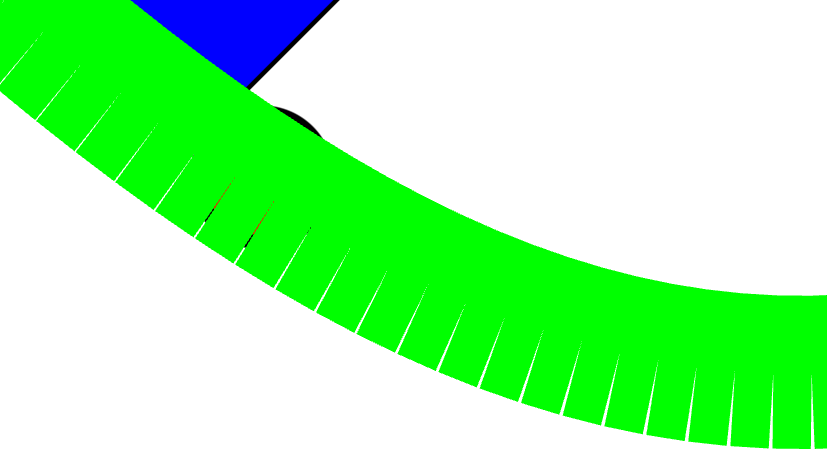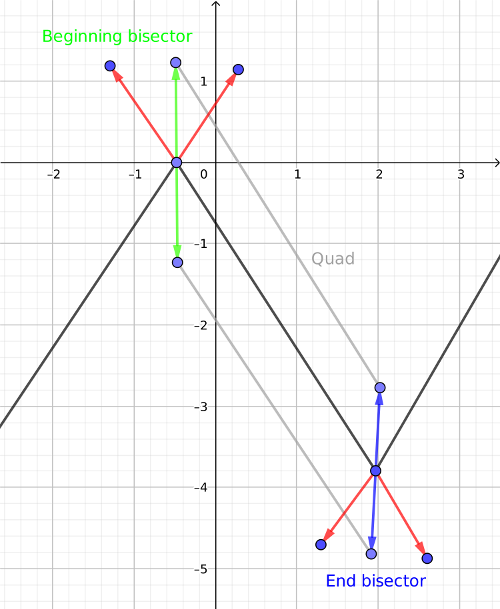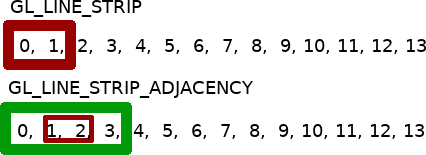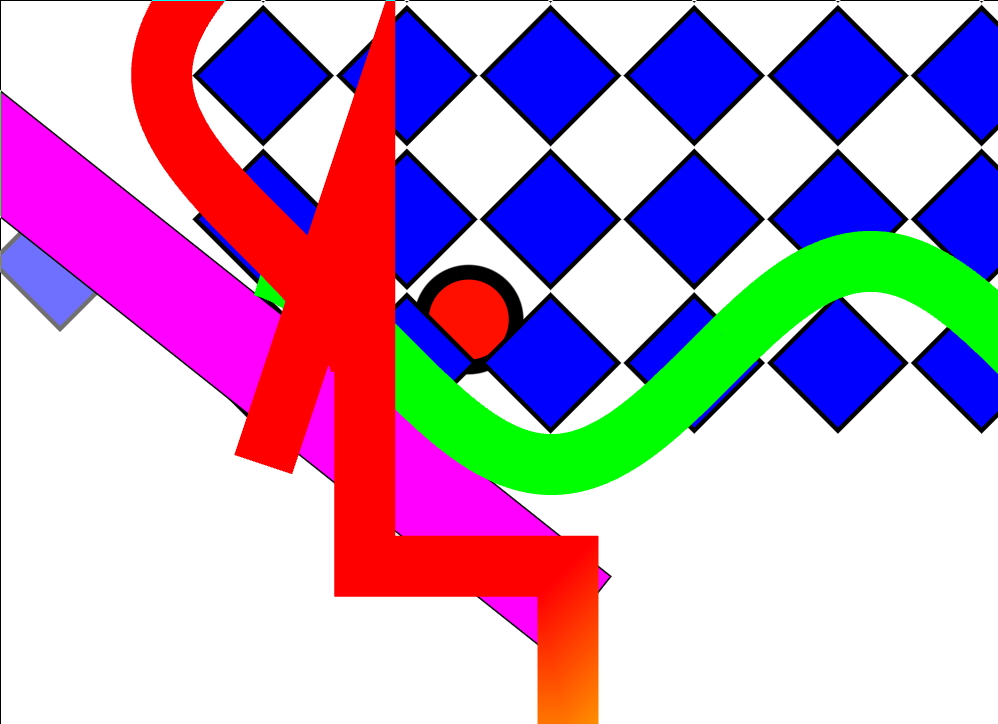# Drawing thick lines in OpenGL with geometry shader

## 2018/11/09

I need a way to render lines for the new renderer I mentioned in a previous post. OpenGL has builtin GL_LINES primitive, but that feature seems to be very unreliable, especially if you need it to render lines thicker than 1 pixel.

Instead I decided to write my own implementation of that feature. The easiest way I came up with was by using geometry shader. Basically geometry shader gets line segments as input and then outputs a triangle strip (quad) for each of them.

One naive implementation would be to use glDrawArrays(GL_LINE_STRIP, …) and then generate a quad like this:

``````vec3 start = gl_in.gl_Position.xyz; // in position in world space
vec3 end = gl_in.gl_Position.xyz;
vec3 lhs = cross(normalize(end-start), vec3(0.0, 0.0, -1.0)); // second argument is plane normal, in this case lines are on XY plane

gl_Position = _ModelToClip * _vec4(start+lhs, 1.0);
EmitVertex();
gl_Position = _ModelToClip * _vec4(start-lhs, 1.0);
EmitVertex();
gl_Position = _ModelToClip * _vec4(end+lhs, 1.0);
EmitVertex();
gl_Position = _ModelToClip * _vec4(end-lhs, 1.0);
EmitVertex();
EndPrimitive();``````

The result looks something like this:If you think about it then it makes perfect sense. Each line segment has different direction, thus the outer edges will not be continuous.
Instead of the lhs vector being perpendicular to the line segment it should be the bisector of the corner formed by adjacent line segments. That bisector vector is where the outer edges of 2 line segments meet up. Thus that vector is shared between beginning of one line segment and end of another one. At the beginning of the line section it’s formed by current line segment and the previous one, at the end its the current line segment and the next one. An easy way to get the bisector would be to take unit length line segment (or their extension) vectors and then lerp to the middle point. It should be equivalent to (a+b)*0.5 for each vector component.Now we know how to do it, but we don’t have enough information in the geometry shader. All the geometry shader knows about right now is the current line segment. Thus we need to use OpenGL 3.2 feature GL_LINE_STRIP_ADJACENCY.
Normally the current line segment in GL_LINE_STRIP is like a sliding window of 2 vertices (start, end), for GL_LINE_STRIP_ADJACENCY it’s a sliding window of 4 vertices (previous, start, end, next). Good programmer as you are, you will immediately notice that it would cause a buffer underflow at index 0 and a buffer overflow at index n-1. That’s why OpenGL will start from index 1 instead of 0 and end at n-2.
This means that we will be missing the first and the last line segment. There is 2 ways I can think of to get around it. First you could duplicate the last and first indices in your index buffer. As a second option you could add an extra vertex to both ends, mirroring the first/last line segment. The first option would require a special case in geometry shader and editing the index buffer. The second one would require editing both index and vertex buffer but no special case in the shader. The second option could also potentially deal with cases the first one can’t. For example line loops - with those extra 2 vertices you could make sure that the ends line up properly. I still went with the first one for now.Here’s what my shaders look like:

``````#version 330 core
layout(location = 0) in vec4 position;
layout(location = 1) in vec4 color;
out vec4 v_color;
out vec3 v_worldPos;
void main() {
gl_Position = position;
v_color = color;
}``````

``````#version 330 core
layout (triangle_strip, max_vertices = 4) out;
in vec4 v_color;
out vec4 g_color;

uniform mat4 _ModelToClip;
uniform float _LineWidth;

void main() {
vec3 prev = gl_in.gl_Position.xyz;
vec3 start = gl_in.gl_Position.xyz;
vec3 end = gl_in.gl_Position.xyz;
vec3 next = gl_in.gl_Position.xyz;

vec3 lhs = cross(normalize(end-start), vec3(0.0, 0.0, -1.0));

// is previous line segment a zero vector?
bool colStart = length(start-prev) < 0.0001; // 0.0001 is arbitrary epsilon
// is next line segment a zero vector?
bool colEnd = length(end-next) < 0.0001;

vec3 a = normalize(start-prev);
vec3 b = normalize(start-end);
vec3 c = (a+b)*0.5;
vec3 startLhs = normalize(c) * sign(dot(c, lhs));
a = normalize(end-start);
b = normalize(end-next);
c = (a+b)*0.5;
vec3 endLhs = normalize(c) * sign(dot(c, lhs));

if(colStart)
startLhs = lhs;
if(colEnd)
endLhs = lhs;

float startInvScale = dot(startLhs, lhs);
float endInvScale = dot(endLhs, lhs);

startLhs *= _LineWidth*0.5;
endLhs *= _LineWidth*0.5;

gl_Position = _ModelToClip*vec4(start+startLhs/startInvScale, 1.0);
g_color = v_color;
EmitVertex();
gl_Position = _ModelToClip*vec4(start-startLhs/startInvScale, 1.0);
EmitVertex();
gl_Position = _ModelToClip*vec4(end+endLhs/endInvScale, 1.0);
g_color = v_color;
EmitVertex();
gl_Position = _ModelToClip*vec4(end-endLhs/endInvScale, 1.0);
EmitVertex();
EndPrimitive();
}``````
End result: